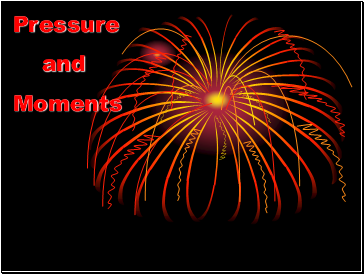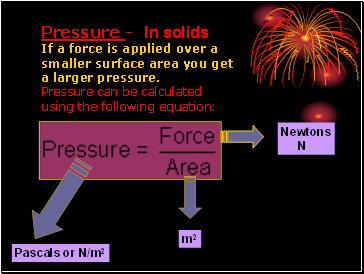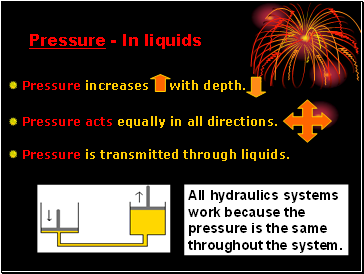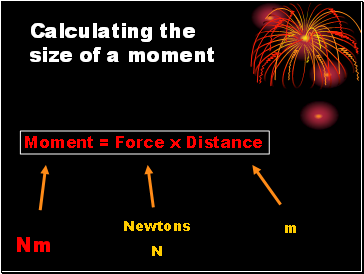# Pressure and momentsPage 1

#### WATCH ALL SLIDES

Slide 1## Pressure and Moments

Slide 2Pressure - In solids

If a force is applied over a smaller surface area you get a larger pressure.

Pressure can be calculated using the following equation:

Newtons

N

Pascals or N/m2

m2

Slide 3## Pressure - In liquids

All hydraulics systems work because the pressure is the same throughout the system.

Slide 4## Moments

Moments make things turn or rotate.

They are caused by forces but are not forces themselves.

The moment will be bigger if:

The force causing the turning effect is bigger.

The force is further from the pivot.

Slide 5Calculating the size of a moment

Moment = Force x Distance

Newtons

N

m

Nm

Slide 6## Balanced

Anti-clockwise Moment = Clockwise Moment

Net moment is zero